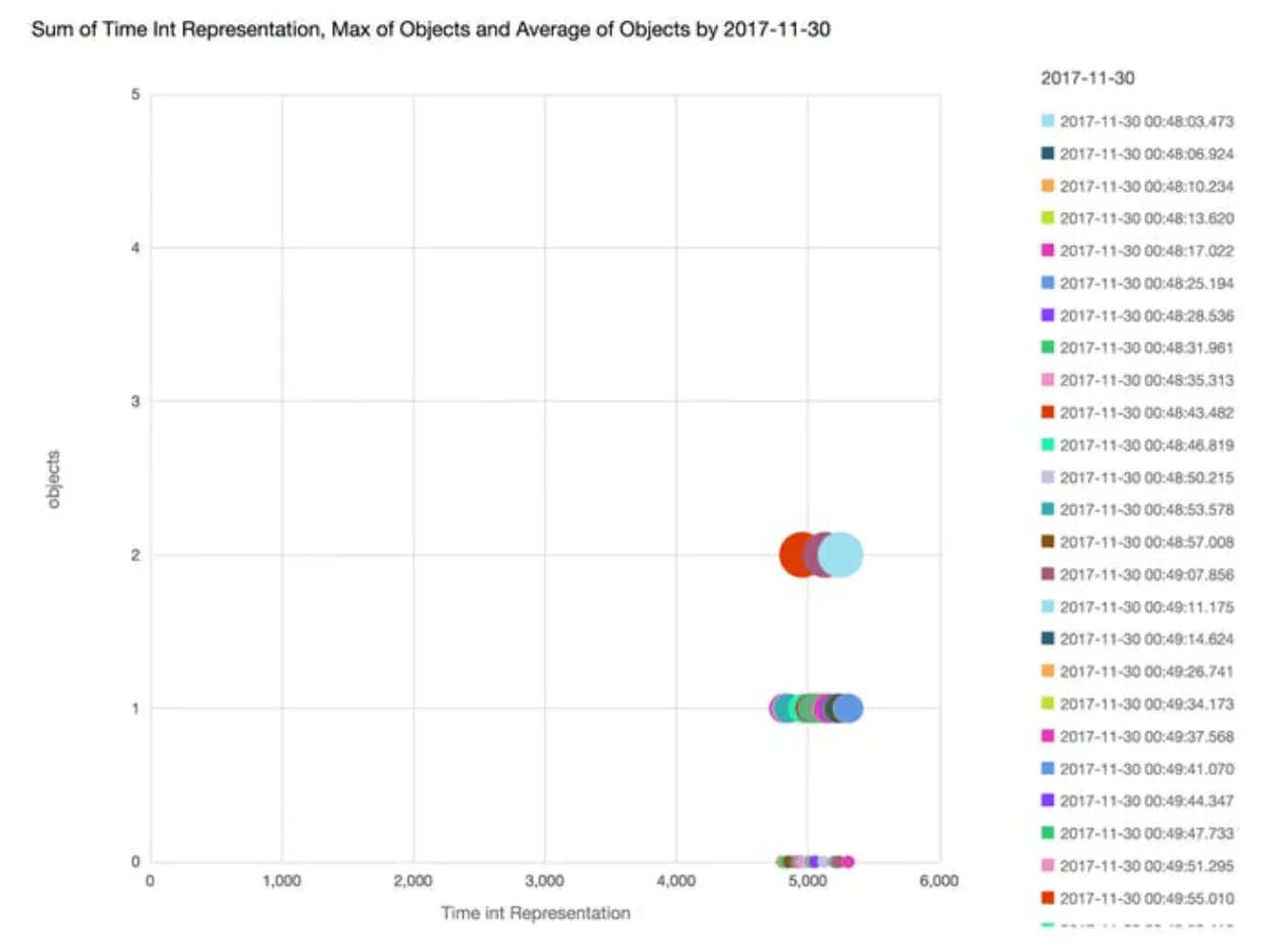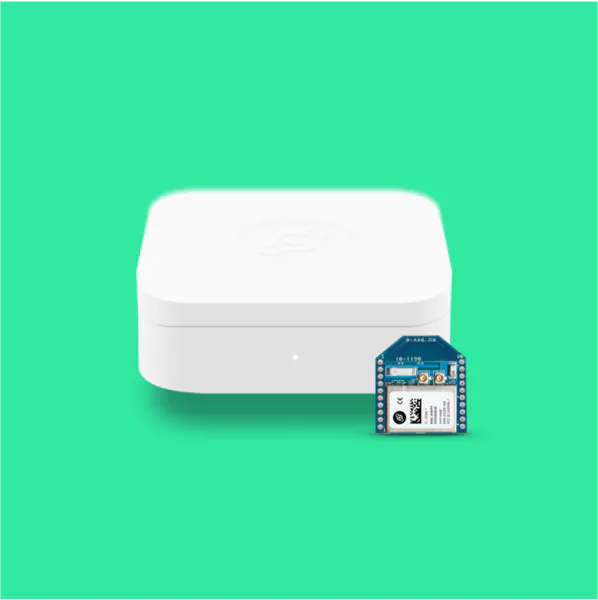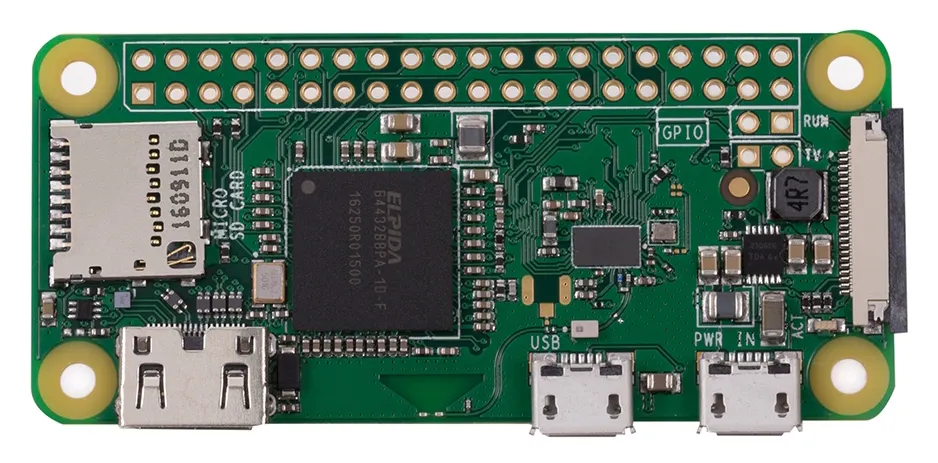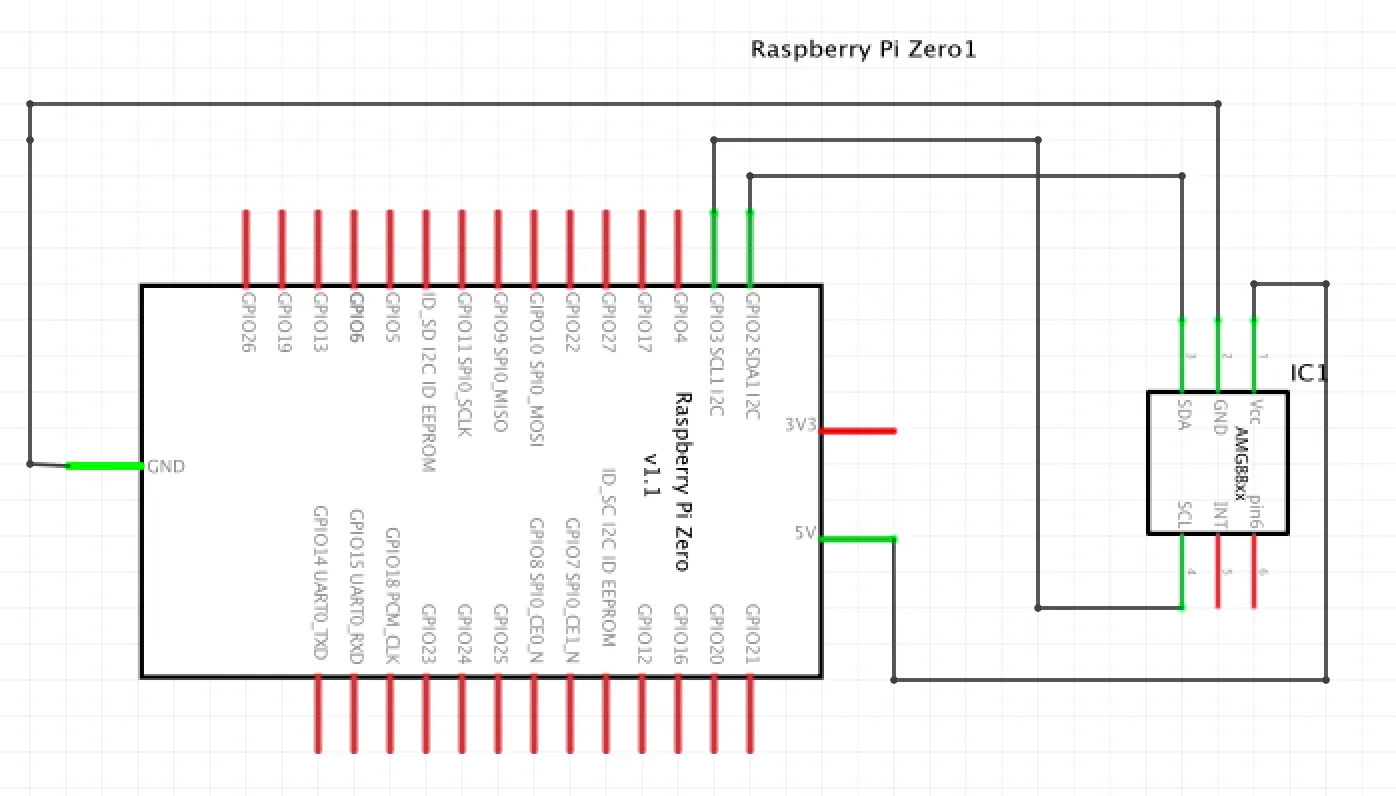# People Counting with Helium, Grid-EYE, and Raspberry Pi

A simple way to identify objects in a room using the Grid-EYE, a Raspberry Pi, OpenCV, and the Helium Network.

IntermediateFull instructions provided1 hour10,708## Things used in this project

### Hardware components

 Panasonic AMG8833 Grid-EYE
×1Helium Starter Kit (LEGACY)
×1
×1Raspberry Pi 3 Model B
×1Raspberry Pi Zero Wireless
×1

### Software apps and online servicesOpenCV

## Schematics

### Extracted AWS IoT Data

Here is a short result of the Grid-Eye scanning a room with a 30 degree celsius or higher threshold

### Raspberry Pi Zero Schematic## Code

### R Pi GridEye

Python
Connecting the Pi to the Grid-Eye and transmitting to Helium Channel code
```import numpy as np
import math
import time
from matplotlib import pyplot as plt
from scipy.interpolate import griddata
import cv2
from helium_client import Helium

helium = Helium("YOUR SERIAL PORT HERE")
helium.connect()

channel = helium.create_channel("YOUR CHANNEL NAME HERE")

# Access an instance of Configuration
config = channel.config()
# Start sensor

active = True

while(1):
if active == True:
# Read pixels, convert them to values between 0 and 1, map them to an 8x8 grid
pixmax = max(pixels)
pixels = [x / pixmax for x in pixels]
points = [(math.floor(ix / 8), (ix % 8)) for ix in range(0, 64)]
grid_x, grid_y = np.mgrid[0:7:32j, 0:7:32j]

# bicubic interpolation of 8x8 grid to make a 32x32 grid
bicubic = griddata(points, pixels, (grid_x, grid_y), method='cubic')
image = np.array(bicubic)
image = np.reshape(image, (32, 32))
print(image)
plt.imsave('color_img.jpg', image)

img = cv2.bitwise_not(img)

# Setup SimpleBlobDetector parameters.
params = cv2.SimpleBlobDetector_Params()

# Change thresholds
params.minThreshold = 10
params.maxThreshold = 255

# Filter by Area.
params.filterByArea = True
params.minArea = 5

# Filter by Circularity
params.filterByCircularity = True
params.minCircularity = 0.1

# Filter by Convexity
params.filterByConvexity = False
params.minConvexity = 0.87

# Filter by Inertia
params.filterByInertia = False
params.minInertiaRatio = 0.01

# Set up the detector with default parameters.
detector = cv2.SimpleBlobDetector_create(params)

# Detect blobs.
keypoints = detector.detect(img)

for i in range (0, len(keypoints)):
x = keypoints[i].pt
y = keypoints[i].pt
print(x, y)

# Get the channel setting for interval_ms
interval = config.get("channel.interval_ms")
interval = config.get("channel.active")
# Report the device having set interval_ms
config.set("channel.interval_ms", interval)
config.set("channel.active", active)
channel.send(str(len(keypoints)))
time.sleep(interval)
# time.sleep(5)

else:
print("idle")
channel.send("Idle")
time.sleep(5)
interval = config.get("channel.active")
config.set("channel.active", active)
```

Add this through the Arduino library manager or through the public repository to test and operate the AMG88xx version Grid-EYE boards from Adafruit.

## Credits

### Robert Keith

1 project • 3 followers

### Mark Phillips

2 projects • 13 followers
Director of Customer Success at Helium